# Hexacontagon facts for kids

Kids Encyclopedia Facts
Regular hexacontagon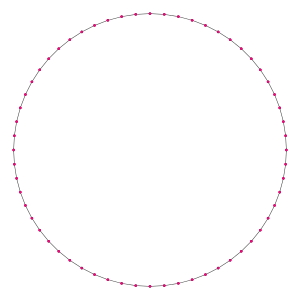A regular hexacontagon
Type Regular polygon
Edges and vertices 60
Schläfli symbol {60}, t{30}, tt{15}
Coxeter diagram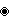Symmetry group Dihedral (D60), order 2×60
Internal angle (degrees) 174°
Dual polygon Self
Properties Convex, cyclic, equilateral, isogonal, isotoxal

A hexacontagon or 60-gon is a shape with 60 sides and 60 corners.

## Regular hexacontagon

A regular hexacontagon is represented by Schläfli symbol {60} and also can be constructed as a truncated triacontagon, t{30}, or a twice-truncated pentadecagon, tt{15}. A truncated hexacontagon, t{60}, is a 120-gon, {120}.

One interior angle in a regular hexacontagon is 174°, meaning that one exterior angle would be 6°.

### Area

The area of a regular hexacontagon is (with t = edge length)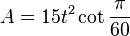$A = 15t^2 \cot \frac{\pi}{60}$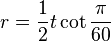$r = \frac{1}{2}t \cot \frac{\pi}{60}$

The circumradius of a regular hexacontagon is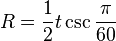$R = \frac{1}{2}t \csc \frac{\pi}{60}$

This means that the trigonometric functions of π/60 can be expressed in radicals.

### Constructible

Since 60 = 22 × 3 × 5, a regular hexacontagon is constructible using a compass and straightedge. As a truncated triacontagon, it can be constructed by an edge-bisection of a regular triacontagon.

## Dissection

Coxeter states that every zonogon (a 2m-gon whose opposite sides are parallel and of equal length) can be dissected into m(m-1)/2 parallelograms.

In particular this is true for regular polygons with evenly many sides, in which case the parallelograms are all rhombi. For the regular hexacontagon, m=30, and it can be divided into 435: 15 squares and 14 sets of 30 rhombs. This decomposition is based on a Petrie polygon projection of a 30-cube.

## Images for kidsHexacontagon Facts for Kids. Kiddle Encyclopedia.